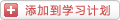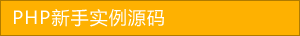﻿ PHP排序算法之冒泡排序(Bubble Sort)实现方法详解 - php应用 - PHP粉丝网

# PHP排序算法之冒泡排序(Bubble Sort)实现方法详解

##### 发布:smiling　来源: PHP粉丝网 　添加日期：2021-09-11 10:50:36　浏览: 评论：0

1. //这里使用了类型提示（type hint） array，不熟悉或者不习惯的同学大可去掉，不影响运算结果
2. function MySort(array &\$arr){
3.   \$length = count(\$arr);
4.   for(\$i = 0;\$i < \$length - 1;\$i ++){
5.     for(\$j = \$i + 1;\$j < \$length;\$j ++){
6.       //将小的关键字放前面
7.       if(\$arr[\$i] > \$arr[\$j]){
8.         \$temp = \$arr[\$i];
9.         \$arr[\$i] = \$arr[\$j];
10.         \$arr[\$j] = \$temp
11.       }
12.     }
13.   }
14. \$arr = array(9,1,5,8,3,7,4,6,2);
15. MySort(\$arr);
16. print_r(\$arr);

1.比较相邻的元素。如果第一个比第二个大，就交换他们两个。

2.对每一对相邻元素作同样的工作，从开始第一对到结尾的最后一对，在这一点，最后的元素应该会是最大的数。

3.针对所有的元素重复以上的步骤，除了最后一个。

4.持续每次对越来越少的元素重复上面的步骤，直到没有任何一对数字需要比较。

1. //交换方法
2. function swap(array &\$arr,\$a,\$b){
3.   \$temp = \$arr[\$a];
4.   \$arr[\$a] = \$arr[\$b];
5.   \$arr[\$b] = \$temp
6. //冒泡排序
7. function BubbleSort(array &\$arr){
8.   \$length = count(\$arr);
9.   for(\$i = 0;\$i < \$length - 1;\$i ++){
10.     //从后往前逐层上浮小的元素
11.     for(\$j = \$length - 2;\$j >= \$i;\$j --){
12.       //两两比较相邻记录
13.       if(\$arr[\$j] > \$arr[\$j + 1]){
14.         swap(\$arr,\$j,\$j+1);
15.       }
16.     }
17.   }

《大话数据结构》果然是本好书，还给我们介绍了冒泡排序算法的改进，这里我就直接搬它的陈述：

1. //交换方法
2. function swap(array &\$arr,\$a,\$b){
3.   \$temp = \$arr[\$a];
4.   \$arr[\$a] = \$arr[\$b];
5.   \$arr[\$b] = \$temp
6. /冒泡排序的优化(如果某一次循环的时候没有发生元素的交换，则整个数组已经是有序的了)
7. function BubbleSort1(array &\$arr){
8.   \$length = count(\$arr);
9.   \$flag = TRUE;
10.   for(\$i = 0;(\$i < \$length - 1) && \$flag;\$i ++){
11.     \$flag = FALSE;
12.     for(\$j = \$length - 2;\$j >= \$i;\$j --){
13.       if(\$arr[\$j] > \$arr[\$j + 1]){
14.         swap(\$arr,\$j,\$j+1);
15.         \$flag = TRUE;
16.       }
17.     }
18.   }

### 分享到： document.write('<a href="http://v.t.sina.com.cn/share/share.php?url='+encodeURIComponent(location.href)+'&appkey=3172366919&title='+encodeURIComponent('PHP排序算法之冒泡排序(Bubble Sort)实现方法详解')+'" title="分享到新浪微博" class="t1" target="_blank">&nbsp;</a>'); document.write('<a href="http://www.douban.com/recommend/?url='+encodeURIComponent(location.href)+'&title='+encodeURIComponent('PHP排序算法之冒泡排序(Bubble Sort)实现方法详解')+'" title="分享到豆瓣" class="t2" target="_blank">&nbsp;</a>'); document.write('<a href="http://share.renren.com/share/buttonshare.do?link='+encodeURIComponent(location.href)+'&title='+encodeURIComponent('PHP排序算法之冒泡排序(Bubble Sort)实现方法详解')+'" title="分享到人人" class="t3" target="_blank">&nbsp;</a>'); document.write('<a href="http://www.kaixin001.com/repaste/share.php?rtitle='+encodeURIComponent('PHP排序算法之冒泡排序(Bubble Sort)实现方法详解')+'&rurl='+encodeURIComponent(location.href)+'&rcontent=" title="分享到开心网" class="t4" target="_blank">&nbsp;</a>'); document.write('<a href="http://sns.qzone.qq.com/cgi-bin/qzshare/cgi_qzshare_onekey?url='+encodeURIComponent(location.href)+'" title="分享到QQ空间" class="t5" target="_blank">&nbsp;</a>');